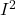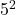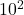## Your new toaster has two separate toasting units, each of which consumes 600 watts of power when it is in use. When you operate one unit, a

Question

Your new toaster has two separate toasting units, each of which consumes 600 watts of power when it is in use. When you operate one unit, a current of 5 amperes flowsthrough the wiring in your home and the wires waste about 1 watt of power handling that current. If you operate both toasting units at once, your toaster consumes 1200 watts and the current flowing through the wiring in your home doubles to 10 amperes. How much power will the wires in your home waste now

in progress 0
3 weeks 2021-08-27T06:15:19+00:00 1 Answers 0 views 0

1.92 Watt lost

Explanation:

Power rating of each toaster = 600 Watts

Current that flows = 5 Amperes

Wasted power = 1 Watt

Voltage of toaster can be gotten from P =R

where I = current

and R = Resistance

600 =x R

R = 600/25 = 24 Ohms.

According to joules loss due to heating of wire

Power loss P ∝R

imputing values,

1 ∝x 24

1 ∝ 600

to remove the proportionality sign, we introduce a constant k

1 = 600k

k = 1/600 = 0.00167

For the case where the current is doubled to 10 ampere, as the power doubles to 1200 W.

The resistance across the wire becomes

1200 =R

R = 1200/100 = 12 Ohms

power loss P = k xR

P = 0.0016 xx 12

P = 1.92 Watt lost